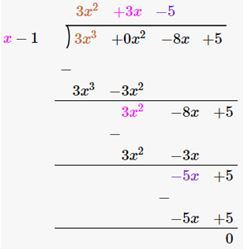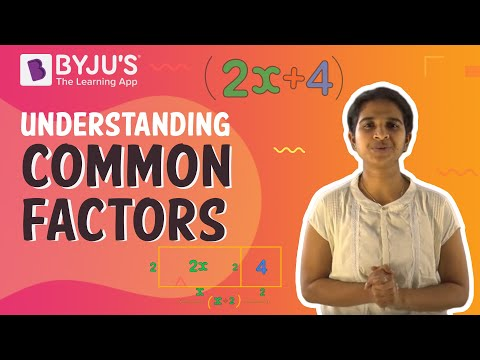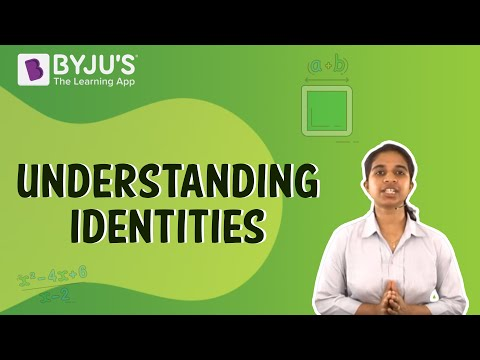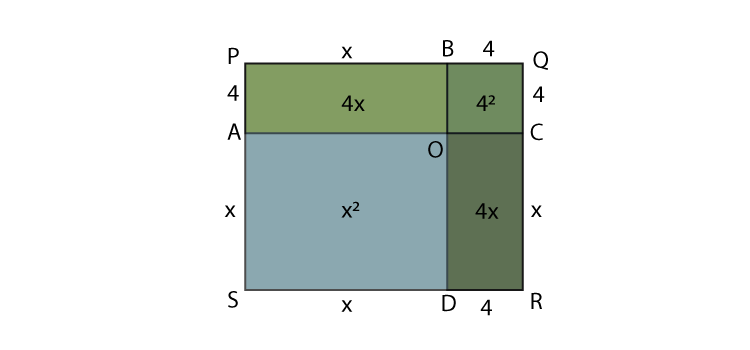# Factorisation Class 8 Notes- Chapter 14

## What are Factors?

An expression can be factorised into the product of its factors. These factors can be algebraic expressions, variables and numbers also.

To know more about Factors, visit here.

### Division of a monomial by another monomial

Division of a monomial by another monomial:

i) Division of 9x2 by 3:

9x2 ÷ 3 = 3(3x2) / 3 = 3x2

ii) Division of 6x2y by 2y:

6x2y÷2y = (6x2)y / 2y = 2y(3x2) / 2y = 3x2

### Division of a polynomial by a monomial

A polynomial 2x3 + 4x2 + 6xis divided by monomial 2x as shown below:

(2x3+4x2+6x)2x = 2x3 / 2x + 4x22x + 6x / 2x = x2+ 2x + 3

### Division of a polynomial by a polynomial

Long division method is used to divide a polynomial by a polynomial.
Example:Division of 3x2 + 3x – 5 by (x – 1) is shown below:To know more about Polynomial Division, visit here.

### Factors of natural numbers

Every number can be expressed in the form of product of prime factors. This is called prime factor form.

Example: Prime factor form of 42 is2 x 3 x 7, where 2, 3 and 7 are factors of 42.

### Algebraic expressions

An algebraic expression is defined as the mathematical expression which consists of variables, numbers, and operations. The values of this expression are the not constant.For example: x + 1, p – q, 3x, 2x+3y, 5a/6b etc.

### Factors of algebraic expressions and factorisation

An irreducible factor is a factor which cannot be expressed further as a product of factors.
Algebraic expressions can be expressed in irreducible form.

To know more about Factorisation of algebraic expression, visit here.

### Method of Common Factors#### Factorisation by common factors

To factorise an algebraic expression, the highest common factors are determined.

Example: Algebraic expression -2y2 + 8y can be written as 2y(-y+4), where 2y is the highest common factor in the expression.

#### Factorisation by regrouping terms

In some algebraic expressions, it is not possible that every term has a common factor. Therefore, to factorise those algebraic expressions, terms having common factors are grouped together.

Example:

= 12a + n – na – 12

= 12a-12+n-na

= 12(a-1)-n(a-1)

= (12-n)(a-1)

(12-n) and (a-1)are factors of the expression 12a+n-na-12

### Method of Identities

#### Algebraic identities

The algebraic equations which are true for all values of variables in them are called algebraic identities.

Some of the identities are,

(a+b)2 = a2+ 2ab + b2

(a-b)2= a2– 2ab + b2

(a+b)(a-b)= a2 – b2

### Factorisation using algebraic identities

Algebraic identities can be used for factorisation

Example: (i)9x2 + 12xy + 4y2

= ( 3x)2 + 2 x 3x x 2y+(2y)2

= (3x+4y)2

(ii)4a2 – b2 = (2a-b)(2a+b)